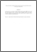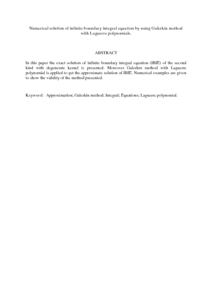# Numerical solution of infinite boundary integral equation by using Galerkin method with Laguerre polynomials.

## Citation

Nik Long, Nik Mohd Asri and Eshkuratov, Zainidin K. and Yaghobifar, Mohammad and Hasan, M. (2008) Numerical solution of infinite boundary integral equation by using Galerkin method with Laguerre polynomials. World Academy of Science, Engineering and Technology, 23. pp. 334-337. ISSN 2070-3724

## Abstract

In this paper the exact solution of infinite boundary integral equation (IBIE) of the second kind with degenerate kernel is presented. Moreover Galerkin method with Laguerre polynomial is applied to get the approximate solution of IBIE. Numerical examples are given to show the validity of the method presented.Preview
PDF (Abstract)
Numerical solution of infinite boundary integral equation by using Galerkin method with Laguerre polynomials.pdfView Item